• ## matlab中巧妙定义分段函数

万次阅读 多人点赞 2019-10-06 19:40:22
学习matlab有一段时间了，可是最近一场考试我发现还有很多简单的问题我都没有学会，比如怎么在matlab中定义分段函数。 最简单的方法当然是使用if~else语句，但是由于很多时候我需要使用函数句柄，将函数视作...
  学习matlab有一段时间了，可是最近在一场考试中我发现还有很多简单的问题我都没有学会，比如怎么在matlab中定义分段函数。

最简单的方法当然是使用if~else语句，但是由于很多时候需要使用函数句柄，将函数视作一个参数，所以if~else定义分段函数并不是一个很好的方法，更好的方法是使用逻辑表达式：

[举例]

表示这个分段函数

[方法]

编写如下代码即可。将函数y分为三个部分，每个部分在自己的范围内时，就乘以1，不在自己范围内时，就乘以0。

x=0:0.01:3;
y=x.*(x<1)+x.^2.*(x>=1&x<2)+(x.^3-4).*(x>=2);
plot(x,y);

十分巧妙！

展开全文• 1个Matlab m文件中定义多个函数运行 编写运行程序会遇到“此上下文不允许函数定义”错误。如果仍希望将全部程序放入一个m文件，并...MATLAB 一个m文件写多个独立的功能函数 MATLAB 一个m文件写...
1个Matlab m文件中定义多个函数运行
编写运行程序会遇到“此上下文中不允许函数定义”错误。如果仍希望将全部程序放入一个m文件中，并可以直接运行出结果，该程序包括主程序及主程序中使用的函数，这些函数内容也会写在同一m文件中，则需要用function main（可以起另一个名字）声明主函数，即主程序，即可运行。
MATLAB 在同一个m文件中写多个独立的功能函数
MATLAB 在同一个m文件中写多个独立的功能函数，从而实现在外部可以直接调用这个文件中的某一个函数。
鉴于MATLAB的函数文件的函数名与文件名要一样，就需要有一个统一的接口来涵盖这些功能函数。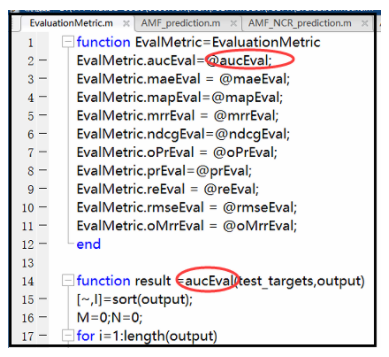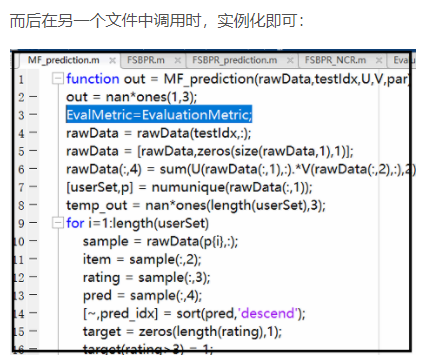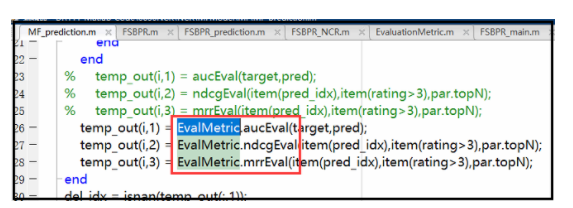展开全文• 如果仍希望将全部程序放入一个m文件，并可以直接运行出结果，该程序包括主程序及主程序使用的函数，这些函数内容也会写同一m文件，则需要用“function main”声明主函数，即主程序，同时也要合理使用全局...
如何在1个Matlab m文件中定义多个函数直接运行

简介

编写运行程序会遇到“此上下文中不允许函数定义”错误。如果仍希望将全部程序放入一个m文件中，并可以直接运行出结果，该程序包括主程序及主程序中使用的函数，这些函数内容也会写在同一m文件中，则需要用“function main”声明主函数，即主程序，同时也要合理使用全局变量，向多个函数传递中间参数值。接下来将用实例介绍，如何定义全局变量，如何编写出可执行的，包含多个自定义函数的程序。

解决方法

clc
clear all;
global a
global b
global c
a=1;
b=2;
c=3;
d=10;
disp(10);

global a
global b
global c
w=(a+b+c)/d;


2.从中可以看到全局变量的使用方法，即先要声明再赋值，最后如果在函数中直接使用，需要在函数体中再次声明该变量为全局定义。

clc
clear all;
%声明全局变量a,b,c
global a
global b
global c
%变量赋值
a=1;
b=2;
c=3;
d=10;

%函数内也要声明全局变量
global a
global b
global c
w=(a+b+c)/d;

3.此时如果点击m程序上的“运行”，则可在结果界面看到此错误，提示不允许这样定义函数，这时可通过声明主函数解决。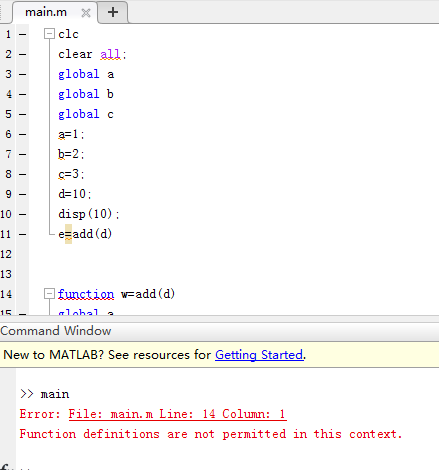4.这里加入如下第一句主函数声明语句后，编译框会自动识别出函数范围，使函数变为可折叠状态，不像之前，无法区分主程序和函数。

%声明函数main(可以起另一个名字)后，就不会出错了
function main
clc
clear all;
global a
global b
global c
a=1;
b=2;
c=3;
d=10;
disp(10);

global a
global b
global c
w=(a+b+c)/d;

5.程序识别出函数后，即可进行折叠，如果一个程序中有较长的主程序和较多函数，即可边编辑程序，边进行折叠，使其看起来更简洁。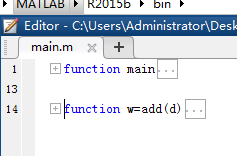6.此时运行后，就没有错误提示，可以直接计算出结果，根据计算公式，可以知道此结果是正确的。这样如何定义全局变量，如何将所有函数放入一个文件，介绍完成。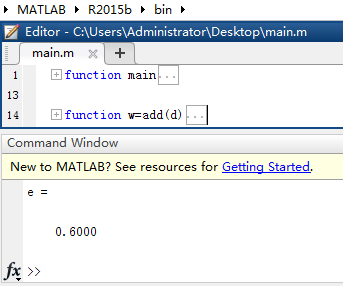另外注意的点

在一个m文件中写多个函数,每个函数都以function开始,结束可以带end,也可以不带（这种情况下,出现另一个function或者到达文件结尾表明前一个function结束）.
第一个函数作为主函数,可以从该m文件外部调用,其它函数都是局部函数,只能被m文件中的函数调用.主函数命名最好和m文件名相同,如果不同,则调用时应该使用m文件名（不带扩展名）.
展开全文• f=@(x)acos(x) 表示 f 为函数句柄，@是定义句柄的运算符。f=@(x)acos(x) 相当于建立了一个函数文件：% f.mfunction y=f(x)y=acos(x);... 匿名函数它是matlab中定义的一种函数形式，出现在matlab中，匿名函数不以...
f=@(x)acos(x)

表示 f 为函数句柄，@是定义句柄的运算符。f=@(x)acos(x) 相当于建立了一个函数文件：% f.mfunction  y=f(x)y=acos(x);

@是匿名函数的意思

函数句柄的使用方法也和函数是一样的。

扩展资料

匿名函数它是matlab中定义的一种函数形式，出现在matlab中，匿名函数不以文件形式驻留在文件夹上；他的生成方式最简捷，可在指令窗或任何函数体内通过指令直接生成。

匿名函数的作用主要是实现自己定义matlab中的函数，从而扩大函数的使用功能

匿名函数的调用方式

匿名函数，顾名思义就是没有名字的函数。上面的函数表达式中的创建，即创建一个匿名函数，并将匿名函数赋值给变量ss，用ss来进行函数的调用，调用的方式就是在变量ss后面加上一对括号()，如果有参数传入的话就是ss(1,2)，这就是匿名函数的一种调用方式。

还有一种匿名函数的调用方式是：使用()将匿名函数括起来，然后后面再加一对小括号（包含参数列表）。

我们再看一下以下一个例子：

在javascript中，是没有块级作用域这种说法的，以上代码的这种方式就是模仿了块级作用域(通常成为私有作用域)，语法如下所示：

(function(){//这里是块级作用域})();

以上代码定义并立即调用了一个匿名函数。经函数声明包含在一对圆括号中，表示它实际上是一个函数表达式。而紧随其后的另一对圆括号会立即调用这个函数。

然而要注意一点：

function(){}();

上面的代码是错误的，因为Javascript将function关键字当作一个函数声明的开始，而函数声明后面不能加圆括号，如果你不显示告诉编译器，它会默认生成一个缺少名字的function，并且抛出一个语法错误，因为function声明需要一个名字。

但是，即便你为上面那个错误的代码加上一个名字，他也会提示语法错误，只不过和上面的原因不一样。在一个表达式后面加上括号()，该表达式会立即执行，但是在一个语句后面加上括号()，是完全不一样的意思，他的只是分组操作符。

参考资料

百度百科——匿名函数

展开全文• 平常我们在matlab中自行编写一个函数，编译器规定函数名和文件名必须一致，这样如果我们需要定义多个函数，就需要编写多个.m文件，数量一多容易混淆。 为了实行MATLAB 一个m文件写多个独立的功能函数，从而...
• ## matlab如何在文中定义函数

万次阅读 多人点赞 2017-01-04 14:12:54
matlab如何文中定义函数 通常我们多是将函数单独编写单个的m文件，再于主程序调用。但是，有时候觉得程序太小，直接程序末尾编写函数，又会收到“此上下文不允许函数定义”的错误提示。那么，matlab...
• 1.m文件，function y=f(x) %函数的声明 y=x^2就是建立了一个y=x2y=x^2的函数，f是函数的名称，m文件的名称必须是f.m. 如果返回值不止一个，则可以： function [x,y,z] = f(a,b) x=a+b y=a-b z=a*b一个M文件...
• ## matlab函数定义

千次阅读 2019-06-11 14:26:22
matlab定义函数，和C语言类似，定义一个从华氏度到摄氏度的函数如下 function D =F2C() for i=0: 10 F = input('==>Temperature in F:','s');%input返回的是一个字符串，需要str2num转化成数字 if isempty(F) ...
• ## MATLAB02:结构化编程和函数定义

万次阅读 多人点赞 2019-11-13 13:43:31
文章目录MATLAB02:结构化编程和函数定义结构化编程流程控制语句和逻辑运算符流程控制语句示例使用循环语句应尽量预先分配内存空间 MATLAB02:结构化编程和函数定义 结构化编程 流程控制语句和逻辑运算符 与大多数编程...
• matlab作为一个大型的计算软件，里面有许多对数组的操作，所以数组的定义和数组的操作是一个必不可少的部分。 1 数组的定义 在matlab中对数组的定义较为灵活，因为特殊矩阵较多，所以有许多特定的定义方法。比较...
• 是素数返回1，不是素数...实现方式是用要求的数从2到它本身减依次相除如果都不能整除那么就是素数，否则不是。 function p=panduanpf(a) for i=2:a-1 if 0==rem(a,i) 0 break; elseif i==a-1 1 end end ...
• 在matlab中定义一个分段函数，然后对其定积分，举例如下： syms x y=1*(x(-2) & x>=(-1))+2*(x(-1))+3*(x<=1 & x>0); int(y,x,-1,1) 结果报错如下： 错误使用 symengine The integrand is invalid. ...x
• ## matlab函数定义详解

万次阅读 2014-06-28 16:23:49
但凡经过编程熏陶的人，学习其他一门陌生... 数学定义一个函数，我们需要明确定义域、值域、表达式。在matlab中，则对应为输入参数，输出参数，函数体。实际上，matlab支持多输入多输出，函数的返回值可以为一
• function [dev] = get_deviations(ws) #这定义函数 x = length(ws) ; sum = 0 ; for i = 1:x sum = sum + ws(i) ; end ave = sum/x ; for i = 1:x dev(i) = ws(i) - ave ; end for i = 1:x #使用定义函数 ...
• 在matlab中，M文件分为脚本文件和函数文件。如果M文件的第一个可执行语句以function开头，那这个M文件就是函数文件。函数文件内定义的变量为局部变量，只在函数文件内部起作用，当函数文件执行完后，这些内部变量将...
• matlab支持多输入多输出，函数的返回值可以为一个向量，矩阵，或者多个元素。为了让函数可以调用，需要对函数进行命名。 function [输出变量] = 函数名称(输入变量） 即包含： 输入参数 输出参数 函数名 注释 ...
• ## matlab函数定义格式

万次阅读 2012-03-30 21:24:15
总结matlab中函数定义的一些内容： ...在matlab中应该做成M文件,文件名要和你文件里的function后面的函数名一致 File新建一个M-file M-file里编辑函数 格式为: function [输出实参表]=函数名(输入实function file c
• %功能描述：定义x为0到2pi之间的组向量，且x向量相邻两值之间的增量为pi/1000，定义y向量的值为x向量的sin值， %绘制出函数图像 x = 0:pi/1000:2*pi; %%定义x为0到2pi之间的组向量，且x向量相邻两值...
• ## Matlab定义子函数

千次阅读 2015-06-30 11:31:13
上篇博客介绍了在Matlab中自定义简单函数的方法，本篇博客将介绍定义子函数的方法。本文承接上篇博客的例子，即随机生成一个3行4列的矩阵，矩阵的元素设定上下限为（low，high），并返回矩阵所有元素的和。.m文件...
• matlab帮助系统的说明： Functions The main difference between a script and a function is thata function accepts input from and returns outputfunction variables nested
• matlab中Function函数的使用并写一个分段函数
• function re=add_multi(a,b,c,d) ...% nargin 表示参数数 if nargin==4 re=a+b+c+d; elseif nargin==3 re=a+b+c; elseif nargin==2 re=a+b; else error('输入有错啊！'); end add_multi(1,2,5) add_multi(3,...

# 在matlab中定义一个函数matlab 订阅